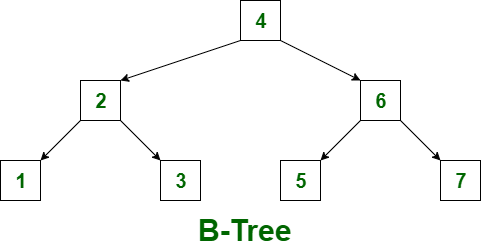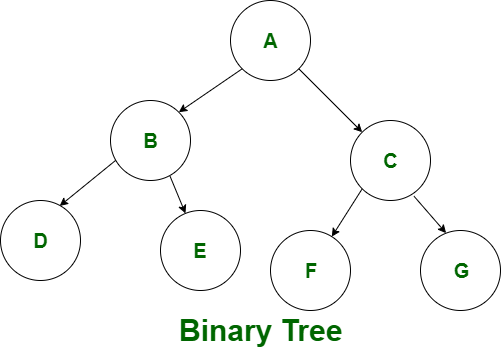# Difference between Binary tree and B-tree

B-Tree :
B-Tree is known as self-balancing tree as its nodes are sorted in inorder traversal. Unlike binary tree, in B-tree, a node can have more than two children. B-tree has a height of logM N (Where ‘M’ is the order of tree and N is the number of nodes). And the height is adjusts automatically at each update. In the B-tree data is sorted in a specific order, with the lowest value on the left and the highest value on the right. To insert the data or key in B-tree is more complicated than binary tree.

There are some conditions that must be hold by the B-Tree:

• All the leaf nodes of the B-tree must be at the same level.
• Above the leaf nodes of the B-tree, there should be no empty sub-trees.
• B- tree’s height should lie as low as possible.Binary Tree :
Binary tree is the special type of general tree. Unlike B-tree, in a binary tree a node can have at most two nodes. In a binary tree, there is a limitation on the degree of a node because the nodes in a binary tree can’t have more than two child node(or degree two). The topmost node of a binary tree is called root node and there are mainly two subtrees one is left-subtree and another is right-sub-tree. Unlike the general tree, the binary tree can be empty. Like B-tree, binary tree can also be sorted in inorder traversal. But it can also be sorted in preorder as well as postorder. In binary tree, data insertion is not complicated than B-tree.Let’s see the difference between B-tree and Binary tree:

S.NO B-tree Binary tree
1. In a B-tree, a node can have maximum ‘M'(‘M’ is the order of the tree) number of child nodes. While in binary tree, a node can have maximum two child nodes or sub-trees.
2. B-tree is called as sorted tree as its nodes are sorted in inorder traversal. While binary tree is not a sorted tree. It can be sorted in inorder, preorder or postorder traversal.
3. B-tree has a height of logM N (Where ‘M’ is the order of tree and N is the number of nodes). While binary tree has a height of log2 N(Where N is the number of nodes).
4. B-Tree is performed when the data is loaded in the disk. Unlike B-tree, binary tree is performed when the data is loaded in the RAM(faster memory).
5. B-tree is used in DBMS(code indexing, etc). While binary tree is used in Huffman coding and Code optimization and many others.
6. To insert the data or key in B-tree is more complicated than binary tree. While in binary tree, data insertion is not complicated than B-tree.

Don’t stop now and take your learning to the next level. Learn all the important concepts of Data Structures and Algorithms with the help of the most trusted course: DSA Self Paced. Become industry ready at a student-friendly price.

My Personal Notes arrow_drop_upCheck out this Author's contributed articles.

If you like GeeksforGeeks and would like to contribute, you can also write an article using contribute.geeksforgeeks.org or mail your article to contribute@geeksforgeeks.org. See your article appearing on the GeeksforGeeks main page and help other Geeks.

Please Improve this article if you find anything incorrect by clicking on the "Improve Article" button below.

Article Tags :
Practice Tags :

1

Please write to us at contribute@geeksforgeeks.org to report any issue with the above content.